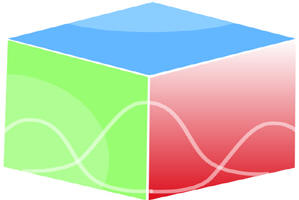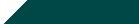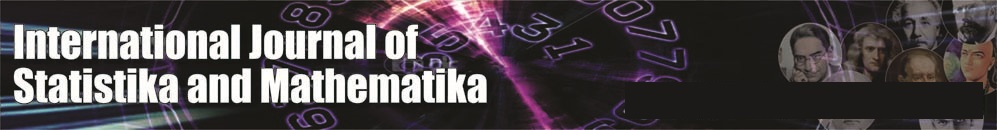``Home| Journals | Statistics Online Expert | About Us | Contact UsUntitled Document

[

Cost-benefit analysis of two-unit cold standby system under the influence of steep high temperatures and human error

Ashok Kumar Saini*

*Associate Professor, Department of Mathematics, BLJS College, Tosham, Bhiwani, Haryana, INDIA.

Email: [email protected]

Research Article

Abstract               Introduction: A large number of papers on a two unit standby redundant systems have appeared because of the large number of parameters governing system behavior and the complex environment with uncertainties. In this paper we have taken open failure mode which is caused due to steep high temperatures (SH temp.) and other failure mode due to human error. When the main unit fails then cold standby system becomes operative. SH temp. cannot occur simultaneously in both the units and after failure the unit undergoes very costly repair facility immediately. Applying the regenerative point technique with renewal process theory the various reliability parameters of interest and profit analysis have been evaluated.

Keyword: Cost-benefit, steep high temperature.

INTRODUCTION

In this paper we have taken open failure mode which is caused due to SH temp. and other failure due to human errors. When the main operative unit fails then cold standby system becomes operative. SH temp. Cannot occur simultaneously in both the units and after failure the unit undergoes repair facility of very high cost in case of open failure mode immediately. Failure due to human error may be destructive. It may be due to repairman’s carelessness or he may be unskilled. The repair is done on the basis of first fail first repaired.

ASSUMPTIONS

1. The failure time distributions are general F1 (t) and F2 (t) and repair is of two types -Type -I, Type-II with repair time distributions as G 1(t) and G 2(t) respectively.
2. The repair starts immediately upon failure of units.
3. The switches are perfect and instantaneous.
4. All random variables are mutually independent.
5. When both the units fail, we give priority to operative unit for repair.
6. The failure of open mode Is due to some human errors.
7. Repairs are perfect and failure of a unit is detected immediately and perfectly.
8. The system is down when both the units are non-operative.
9. After repair, the unit’s works like a new one.

SYMBOLS FOR STATES OF THE SYSTEM

F1(t), F2 (t) - failure time distribution due to SH temp. Mode and open failure mode

G1(t), G2(t) – repair time distribution due to Type -I, Type-II respectively

Superscripts O, CS, SHTF, OF

Operative, Cold Standby, Steep high temp. failure mode, open failure mode due to human error respectively

Subscripts nsht, sht, he, ur, wr, uR

No steep high temp., Steep high temp., human error, under repair, waiting for repair, under repair continued from previous state respectively

Up states: 0, 1, 2;

Down states: 3,4,5,6

Regeneration point: 0, 1, 2

States of the System

0(Onsht, CSnsht)

One unit is operative and the other unit is cold standby and there are no SH temp. in both the units.

1(SHTF sht, ur, Onsht)

The operating unit fails due to SH temp. and is under repair immediately of very costly Type- I and standby unit starts operating with no SH temp.

2(OF nsht,he, ur, Onsht)

The operative unit fails due to human error (open failure mode) and undergoes repair of type II and the standby unit becomes operative with no SH temp..

3(SHTF sht,uR, OF nsht, he,wr)

The first unit fails due to SH temp. and under very costly Type-! Repair is continued from state 1 and the other unit fails due to human errors and is waiting for repair of Type -II.

4(SHTF sht,uR, SHTF sht,wr)

The one unit fails due to SH temp. is continues under repair of very costly Type - I from state 1 and the other unit also fails due to SH temp. is waiting for repair of very costly Type- I.

5(OF nsht, he, uR, SHTF sht, wr)

The operating unit fails due to human error (open failure mode) and under repair of Type - II continues from the state 2 and the other unit fails due to SH temp. is waiting for repair of very costly Type- I.

6(OF nsht,he,uR, OF nsht,he,wr)

The operative unit fails due to human error and under repair continues from state 2 of Type –II and the other unit is also failed due to human error and is waiting for repair of Type-II and there is no SH temp.

TRANSITION PROBABILITIES

Simple probabilistic considerations yield the following expressions :

p01 = , p02 =

p10 = , p13 =p11(3)= p11(4)=

p25 = p225= p226 =

clearly

p01 + p02 = 1,

p10 + p13 =(p113 ) + p14 = ( p114) = 1,

p20 + p25 = (p225) + p26 =(p226) = 1                                                                                                                                                                 (1)

And mean sojourn time are

µ0 = E(T) =                                                                                                                                                           (2)

Mean Time To System Failure

Ø0(t) = Q01(t)[s] Ø1(t) + Q02(t)[s] Ø2(t)

Ø1(t) = Q10 (t)[s] Ø0(t) + Q13(t) + Q14(t)

Ø2(t) = Q20 (t)[s] Ø0(t) + Q25(t) + Q26(t) (3-5)

We can regard the failed state as absorbing

Taking Laplace-Stiljes transform of eq.                                                                                                                                   (3-5)

and solving for

ø0*(s)  = N1(s) / D1(s)                                                                                                                                                                      (6)

Where

N1(s) = Q01*[ Q13 * (s) + Q14 * (s) ] + Q02*[ Q25 * (s) + Q26 * (s) ]

D1(s) = 1 - Q01* Q10* - Q02* Q20*

Making use of relations (1) and (2) it can be shown that ø0*(0)  =1, which implies that ø0*(t)  is a proper distribution.

MTSF = E[T] =  (s)   = (D1(0) - N1(0)) / D1 (0)

s=0

= ( +p01  + p02 ) / (1 - p01 p10 - p02 p20 )

where

= 1 + 2, 1= 0 + 3 + 4

++

Availability analysis

Let Mi(t) be the probability of the system having started from state I is up at time t without making any other regenerative state belonging to E. By probabilistic arguments, we have The value of M0(t), M1(t), M2(t) can be found easily.

The point wise availability Ai(t) have the following recursive relations

A0(t) = M0(t) + q01(t)[c]A1(t) + q02(t)[c]A2(t)

A1(t) = M1(t) + q10(t)[c]A0(t) + q113(t)[c]A1(t)+ q114(t)[c]A1(t),

A2(t) = M2(t) + q20(t)[c]A0(t) + [q225(t)[c]+ q226(t)] [c]A2(t)                                                                                                              (7-9)

Taking Laplace Transform of eq. (7-9) and solving for

= N2(s) / D2(s)                                                                                                                                                         (10)

Where

N2(s) =  0(s)(1 -  11(3)(s) -  11(4)(s)) (1-  225(s)-  226(s)) +  (s)1(s)

[ 1-  225(s)-  226(s)] + 02(s)  2(s)(1- 113(s) - 114(s))

D2(s) = (1 -  113(s)-  114(s)) { 1 -  225(s) - 226(s) ) [1-( 01(s)  10 (s))(1-  113(s)-  114(s))]

A0 =  =  =

Using L’ Hospitals rule, we get

A0 =  =                                                                                                                                      (11)

The expected up time of the system in (0,t] is

(t) =  So that                                                                                                                    (12)

The expected down time of the system in (0,t] is

(t) = t- (t) So that                                                                                                     (13)

The expected busy period of the server when there is open failure mode failure in (0,t]

R0(t) = q01(t)[c]R1(t) + q02(t)[c]R 2(t)

R1(t) = S1(t) + q01(t)[c]R1 (t) + [q113(t) + q114(t)[c]R1(t),

R2(t) = q20(t)[c]R0(t) + [q226(t)+q225(t)][c]R2(t)                                                                                                                     (14-16)

Taking Laplace Transform of eq. (14-16) and solving for

= N3(s) / D3(s)                                                                                                                                                           (17)

Where

N 3(s) = 01(s)  1(s) and

D 3(s)= (1 -  113(s)-  114(s)) – 01(s) is already defined.

In the long run, R0 =                                                                                                                                                      (18)

The expected period of the system under SH temp. in (0,t] is

(t) =  So that

The expected Busy period of the server when there is human error in (0,t]

B0 (t) = q01(t)[c]B1(t) + q02(t)[c]B2(t)

B1(t) = q01(t)[c]B1(t) + [q113(t)+ q114(t)] [c]B1(t),

B2(t) = T2(t) + q02(t)[c] B2(t) + [q225(t)+ q226(t)] [c]B2(t)

T2 (t) = e- λ1t G2(t)                                                                                                                                                                              (19- 21)

Taking Laplace Transform of eq. (19-21) and solving for

= N4(s) / D2(s)                                                                                                                                                         (22)

Where

N4(s) = 02(s)  2(s))

And D2 (s) is already defined.

In steady state, B0 =                                                                                                                                                      (23)

The expected busy period of the server for repair in (0,t] is

(t) =  So that                                                                                                                 (24)

The expected number of visits by the repairman for repairing the different units in (0,t]

H0(t) = Q01(t)[s][1+ H1(t)] + Q02(t)[s][1+ H2(t)]

H1(t) = Q10(t)[s]H0(t)] + [Q113(t)+ Q114(t)] [s]H1(t),

H2(t) = Q20(t)[s]H0(t) + [Q225(t) +Q226(t)] [c]H2(t)                                                                                                                                (25-27)

Taking Laplace Transform of eq. (25-27) and solving for

= N6(s) / D3(s)                                                                                                                                                          (28)

In the long run, H0 =                                                                                                                                                      (29)

COST BENEFIT ANALYSIS

The cost-benefit function of the system considering mean up-time, expected busy period of the system under SH temp. when the units stops automatically, expected busy period of the server for repair of unit, expected number of visits by the repairman for unit failure. The expected total cost-benefit incurred in (0,t] is C(t) = Expected total revenue in (0,t] - expected total repair cost in (0,t] due to OF mode

• expected total repair cost due to human error for repairing the units in (0,t ]
• expected busy period of the system under SH temp. when the units automatically stop in (0,t]
• expected number of visits by the repairman for repairing of the units in (0,t]

The expected total cost per unit time in steady state is

C =  =

= K1A0 - K 2R0 - K 3B0 - K 4H0

Where

K1: revenue per unit up-time,

K2: cost per unit time for which the system is under repair of type- I

K3: cost per unit time for which the system is under repair of type-II

K4: cost per visit by the repairman for units repair.

CONCLUSION

After studying the system, we have analysed graphically that when the failure rate, SH temp. Rate increases, the MTSF and steady state availability decreases and the cost function decreased as the failure increases.

REFERENCES

1. Barlow, R.E. and Proschan, F., Mathematical theory of Reliability, 1965; John Wiley, New York.
2. Gnedanke, B.V., Belyayar, Yu.K. and Soloyer, A.D., Mathematical Methods of Relability Theory, 1969 ; Academic Press, New York.
3. Dhillon, B.S. and Natesen, J, Stochastic Anaysis of outdoor Power Systems in fluctuating environment, Microelectron. Reliab.. 1983; 23, 867-881.
4. Goel, L.R., Sharma,G.C. and Gupta, Rakesh Cost Analysis of a Two-Unit standby system with different weather conditions, Microelectron. Reliab., 1985; 25, 665-659.
5. Goel,L.R., Sharma G.C. and Gupta Parveen, Stochastic Behaviour and Profit Anaysis of a redundant system with slow switching device, Microelectron Reliab., 1986; 26, 215-219.